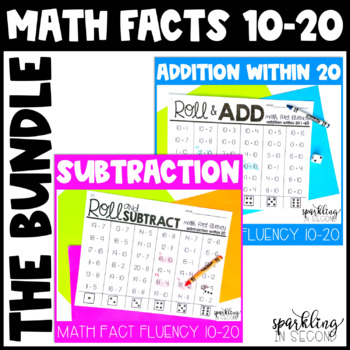digital

# Roll & Add/Subtract within 20-Math Fact Fluency BUNDLE | Distance LearningSubject
Resource Type
Format
Zip (18 MB|110 pages)
Standards
\$7.50
Bundle
List Price:
\$10.00
You Save:
\$2.50
\$7.50
Bundle
List Price:
\$10.00
You Save:
\$2.50The Teacher-Author indicated this resource includes assets from Google Workspace (eg. docs, slides, etc.).

#### Also included in

1. Here is a fun way to practice addition and subtraction math facts! Students roll the dice, pick a column, pick a problem within the column, answer it, and mark it. This is a great way to practice those math facts to automaticity. This also comes with a digital Google Slides version to play online! W
\$15.00
\$20.00
Save \$5.00

### Description

Roll & Add/Subtract within 20-Math Fact Fluency BUNDLE

Here is a fun way to practice math facts within 20! Students roll the dice, pick a column, pick a problem within the column, answer it, and mark it. This is a great way to practice those math facts to automaticity.

This also comes with a digital Google Slides version to play online! Watch the preview video to see how it works.

THIS BUNDLE INCLUDES THE FOLLOWING PACKS (YOU'LL SAVE 20% BY BUYING THE BUNDLE):

SUBTRACTION WITHIN 20

It includes the following fact fluency pages (50 pages totally):

+10 (2)

+9 (2)

+8 (2)

+7 (2)

+6 (2)

doubles (2)

doubles +1 (2)

mixed review addition within 20 (3)

SUBTRACTION:

-10 (2)

-9 (2)

-8 (2)

-7 (2)

-6 (2)

subtract from 20 (2)

subtract from 19 (2)

subtract from 18 (2)

subtract from 17 (2)

subtract from 16 (2)

subtract from 15 (2)

subtract from 14 (2)

subtract from 13 (2)

subtract from 12 (2)

subtract from 11 (2)

mixed review subtraction within 20 (3)

There is an answer key for every Roll page (50 pages + 50 answer keys).

Download the preview for a freebie and to see how to play!

Need more MATH activities? Look HERE!

Want more ROLL activities? Check out my Roll & Reads for reading fluency practice!

***************************************************************************

***************************************************************************

Total Pages
110 pages
Included
Teaching Duration
N/A
Report this Resource to TpT
Reported resources will be reviewed by our team. Report this resource to let us know if this resource violates TpT’s content guidelines.

### Standards

to see state-specific standards (only available in the US).
Fluently add and subtract within 20 using mental strategies. By end of Grade 2, know from memory all sums of two one-digit numbers.
Add and subtract within 20, demonstrating fluency for addition and subtraction within 10. Use strategies such as counting on; making ten (e.g., 8 + 6 = 8 + 2 + 4 = 10 + 4 = 14); decomposing a number leading to a ten (e.g., 13 - 4 = 13 - 3 - 1 = 10 - 1 = 9); using the relationship between addition and subtraction (e.g., knowing that 8 + 4 = 12, one knows 12 - 8 = 4); and creating equivalent but easier or known sums (e.g., adding 6 + 7 by creating the known equivalent 6 + 6 + 1 = 12 + 1 = 13).
Fluently add and subtract within 5.